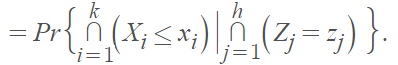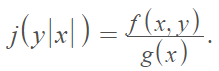# Array Distribution

The array distribution refers to the conditional distribution of independent random variables X1 (given X2, … , Xn). As such, it is often used as a synonym for a conditional distribution (for example, Kotz et al. , Giri & Banerjee , Wadworth & Bryan ).

The Encyclopedia of Statistical Sciences  gives an example of an array distribution of XThe variance of this distribution is called the array variance; if the variance doesn’t depend on X2, … ,Xn , the variation is homoscedastic . Its variance-covariance matrix is called the array-variance-covariance matrix.

## Array Distribution in Applied and Theoretical Statistics

As pointed out above, the array distribution is commonly used as a synonym for conditional distribution. They do mean the same thing, but you’re more likely to see the term array distribution in applied statistics and conditional distribution in theoretical statistics . The array distribution is denoted byYou can think of this as the density of y for a fixed value of x.

References
 Kotz, S. et al. (2000). Continuous Multivariate Distributions, Volume 1 Models and Applications. John Wiley & Sons.

 Giri, P. & Banerjee, J. (2021). Statistical Tools and Techniques. Academic Publishers.

 Wadsworth, G. & Bryan, J. (1960). Introduction to Probability and Random Variables. McGraw-Hill.

 Vidakovic , B. et al. (Eds.) (2005). Encyclopedia of Statistical Sciences, Volume 3. Wiley.

 Johnson, Kotz, and Balakrishnan, (1994), Continuous Univariate Distributions, Volumes I and II, 2nd. Ed., John Wiley and Sons.

 Haight (ed.) (1963). Mathematical Theories of Traffic Flow. Elsevier Science

CITE THIS AS:
Stephanie Glen. "Array Distribution" From StatisticsHowTo.com: Elementary Statistics for the rest of us! https://www.statisticshowto.com/array-distribution/